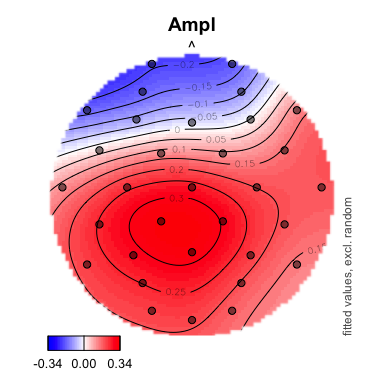# Visual inspection of GAMM models

#### 15 March 2016

In contrast with linear regression models, in nonlinear regression models one cannot interpret the shape of the regression line from the summary. Therefore, visualization is an important tool for interpretating nonlinear regression models. Here a selection of visualization functions is summarized. Detailed information on the functions and examples are found in the help files of each function.

Before summarizing the function, we would like to highlight different concepts

## Example

library(itsadug)
library(mgcv)
data(simdat)
# add missing values to simdat:
simdat[sample(nrow(simdat), 15),]$Y <- NA #### Model We start with a somewhat weird model, for illustration purposes. We would like to include some factorial predictors, random smooths, and a nonlinear interaction. Therefore, the continuous predictor Condition is transformed to a categorical predictor: simdat$Condition <- as.factor(simdat$Condition) # Note: this is really not the best fitting model for the data: m1 <- bam(Y ~ Group * Condition + s(Time) + s(Trial) + ti(Time, Trial) + s(Time, Subject, bs='fs', m=1, k=5), data=simdat, discrete=TRUE) The summary shows two parts: the first summary are the parametric terms, i.e., the ‘linear’ part, and the second summary presents the nonlinear smooths. summary(m1) ## ## Family: gaussian ## Link function: identity ## ## Formula: ## Y ~ Group * Condition + s(Time) + s(Trial) + ti(Time, Trial) + ## s(Time, Subject, bs = "fs", m = 1, k = 5) ## ## Parametric coefficients: ## Estimate Std. Error t value Pr(>|t|) ## (Intercept) 5.510389 0.416535 13.229 < 2e-16 *** ## GroupAdults -1.598632 0.081032 -19.728 < 2e-16 *** ## Condition0 0.219839 0.081032 2.713 0.00667 ** ## Condition1 0.001091 0.081032 0.013 0.98926 ## Condition2 -0.778736 0.081033 -9.610 < 2e-16 *** ## Condition3 -2.117885 0.081040 -26.134 < 2e-16 *** ## Condition4 -4.025739 0.081036 -49.678 < 2e-16 *** ## GroupAdults:Condition0 0.176994 0.114594 1.545 0.12246 ## GroupAdults:Condition1 -0.003609 0.114598 -0.031 0.97487 ## GroupAdults:Condition2 -0.349404 0.114594 -3.049 0.00230 ** ## GroupAdults:Condition3 -1.117771 0.114601 -9.754 < 2e-16 *** ## GroupAdults:Condition4 -1.707418 0.114601 -14.899 < 2e-16 *** ## --- ## Signif. codes: 0 '***' 0.001 '**' 0.01 '*' 0.05 '.' 0.1 ' ' 1 ## ## Approximate significance of smooth terms: ## edf Ref.df F p-value ## s(Time) 8.580 8.685 394.4 <2e-16 *** ## s(Trial) 8.822 8.991 1504.9 <2e-16 *** ## ti(Time,Trial) 12.822 14.561 2878.0 <2e-16 *** ## s(Time,Subject) 148.938 168.000 4902.1 <2e-16 *** ## --- ## Signif. codes: 0 '***' 0.001 '**' 0.01 '*' 0.05 '.' 0.1 ' ' 1 ## ## R-sq.(adj) = 0.966 Deviance explained = 96.6% ## fREML = 1.4607e+05 Scale est. = 2.7425 n = 75585 Note that although the model looks good (deviance explained of 96.62%!), there are some issues with this model, which we won’t address here. #### Function gamtabs With the function gamtabs the summary of the model can be converted to a Latex of HTML table, easy to integrate in a knitr or R Markdown report (see here): gamtabs(m1, type="HTML")  A. parametric coefficients Estimate Std. Error t-value p-value (Intercept) 5.5104 0.4165 13.2291 < 0.0001 GroupAdults -1.5986 0.0810 -19.7284 < 0.0001 Condition0 0.2198 0.0810 2.7130 0.0067 Condition1 0.0011 0.0810 0.0135 0.9893 Condition2 -0.7787 0.0810 -9.6102 < 0.0001 Condition3 -2.1179 0.0810 -26.1340 < 0.0001 Condition4 -4.0257 0.0810 -49.6782 < 0.0001 GroupAdults:Condition0 0.1770 0.1146 1.5445 0.1225 GroupAdults:Condition1 -0.0036 0.1146 -0.0315 0.9749 GroupAdults:Condition2 -0.3494 0.1146 -3.0491 0.0023 GroupAdults:Condition3 -1.1178 0.1146 -9.7536 < 0.0001 GroupAdults:Condition4 -1.7074 0.1146 -14.8988 < 0.0001 B. smooth terms edf Ref.df F-value p-value s(Time) 8.5801 8.6847 394.4193 < 0.0001 s(Trial) 8.8216 8.9905 1504.9066 < 0.0001 ti(Time,Trial) 12.8223 14.5612 2877.9665 < 0.0001 s(Time,Subject) 148.9376 168.0000 4902.1097 < 0.0001 ## Partial versus Summed effects An important distinction in regression model is the distinction between partial effects and summed effects: • partial effects are the isolated effects of one particular predictor or interaction. Only one line of the summary… If you use the function plot for visualizing the model terms, you will see the partial effects. Try plot(m1, all.terms=TRUE, rug=FALSE). • summed effects are the predicted response measures for a certain situation or condition. So all the partial effects that apply to that situation are summed up, including the intercept. If not for all predictors a value is given, the functions automatically select a value for unspecified predictors. More information about these settings are provided in the summary in the console. If you do not see this summary, use the command infoMessages("on"). ## Inspection of summed effects #### Function plot_smooth The function plot_smooth visualizes 1-dimensional nonlinear effects as summed effects. The summary of the first plot below shows that this plot is specifically plotting the summed effect of a certain Subject and a certain Trial for the “Adults” age group. ## Summary: ## * Group : factor; set to the value(s): Adults. ## * Condition : factor; set to the value(s): 2. ## * Time : numeric predictor; with 30 values ranging from 0.000000 to 2000.000000. ## * Trial : numeric predictor; set to the value(s): 0. ## * Subject : factor; set to the value(s): a12. (Might be canceled as random effect, check below.) ## * NOTE : The following random effects columns are canceled: s(Time,Subject) ##  Generally, we would like to generalize over the random effects predictors, such as Subject. We can exclude the random effects from the summed effects plots by the argument rm.ranef=TRUE. Note the labels at the right side of the plot indicate the chosen settings. par(mfrow=c(1,2), cex=1.1) # including random effects: plot_smooth(m1, view="Time", cond=list(Group="Adults")) # excluding random effects: plot_smooth(m1, view="Time", cond=list(Group="Adults"), rm.ranef=TRUE)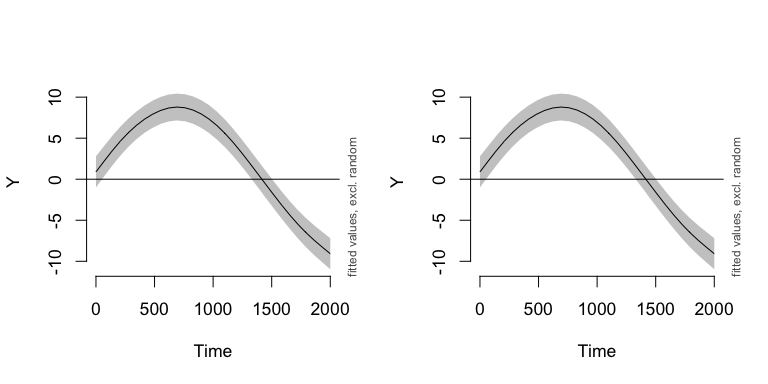The plots below show two different ways for combining the two age groups in one plot: par(mfrow=c(1,2), cex=1.1) # version 1: plot_smooth(m1, view="Time", plot_all="Group", rm.ranef=TRUE, ylim=c(-15,15)) # version 2: plot_smooth(m1, view="Time", cond=list(Group="Adults"), rm.ranef=TRUE, rug=FALSE, col="red", ylim=c(-15,15)) plot_smooth(m1, view="Time", cond=list(Group="Children"), rm.ranef=TRUE, rug=FALSE, col="cyan", add=TRUE)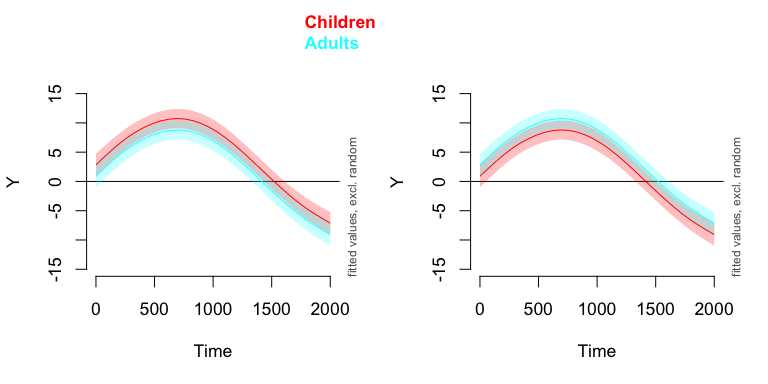#### Function fvisgam Two dimensional surfaces are used for visualizing higher order interactions, such as TimexTrial. Again, the argument rm.ranef=TRUE is used for canceling the random effects. Note the difference in scale on the color legend: par(mfrow=c(1,2), cex=1.1) # including random effects: fvisgam(m1, view=c("Time", "Trial"), cond=list(Group="Adults")) ## Warning in gradientLegend(c(min.z, max.z), n.seg = 3, pos = 0.875, color = ## pal, : Increase right margin to fit labels or decrease the number of decimals, ## see help(gradientLegend). # excluding random effects: fvisgam(m1, view=c("Time", "Trial"), cond=list(Group="Adults"), rm.ranef=TRUE) ## Warning in gradientLegend(c(min.z, max.z), n.seg = 3, pos = 0.875, color = ## pal, : Increase right margin to fit labels or decrease the number of decimals, ## see help(gradientLegend).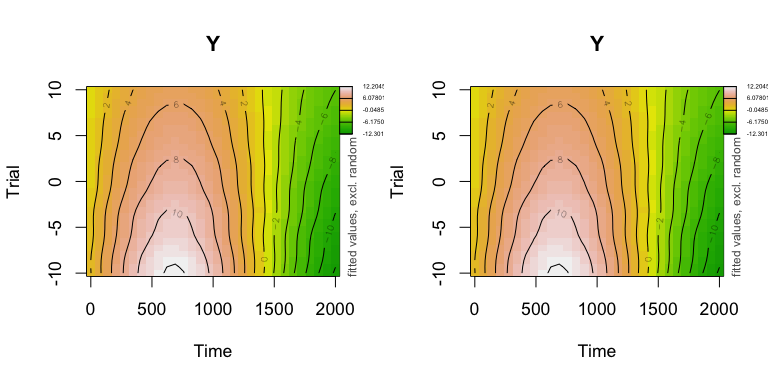Setting the color range to the same scale is important when comparing surfaces: par(mfrow=c(1,2), cex=1.1) # group children: fvisgam(m1, view=c("Time", "Trial"), cond=list(Group="Children"), rm.ranef=TRUE, zlim=c(-15,15), main="Children") ## Warning in gradientLegend(c(min.z, max.z), n.seg = 3, pos = 0.875, color = ## pal, : Increase right margin to fit labels or decrease the number of decimals, ## see help(gradientLegend). # group Adults: fvisgam(m1, view=c("Time", "Trial"), cond=list(Group="Adults"), rm.ranef=TRUE, zlim=c(-15,15), main="Adults") ## Warning in gradientLegend(c(min.z, max.z), n.seg = 3, pos = 0.875, color = ## pal, : Increase right margin to fit labels or decrease the number of decimals, ## see help(gradientLegend).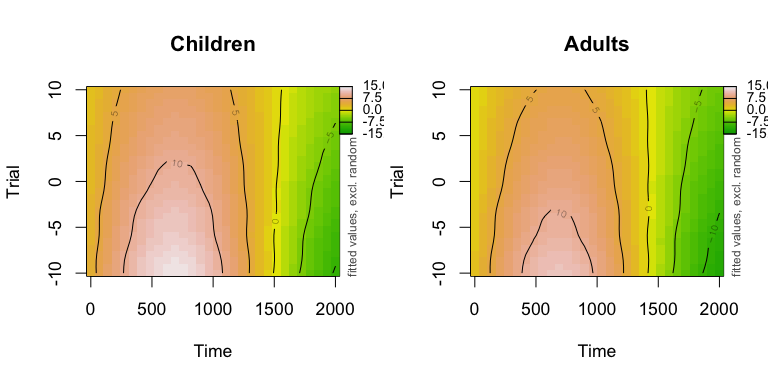#### Function plot_parametric The function plot parametric plots the summed effects of the parametric terms. par(mfrow=c(1,3), cex=1.1) # all: plot_parametric(m1, pred=list(Condition=levels(simdat$Condition),Group=c('Adults', 'Children')), rm.ranef=TRUE)
# children:
plot_parametric(m1,  pred=list(Condition=levels(simdat$Condition)), cond=list(Group="Children"), rm.ranef=TRUE, main="Children") # adults: plot_parametric(m1, pred=list(Condition=levels(simdat$Condition)), cond=list(Group="Adults"), rm.ranef=TRUE, main="Adults")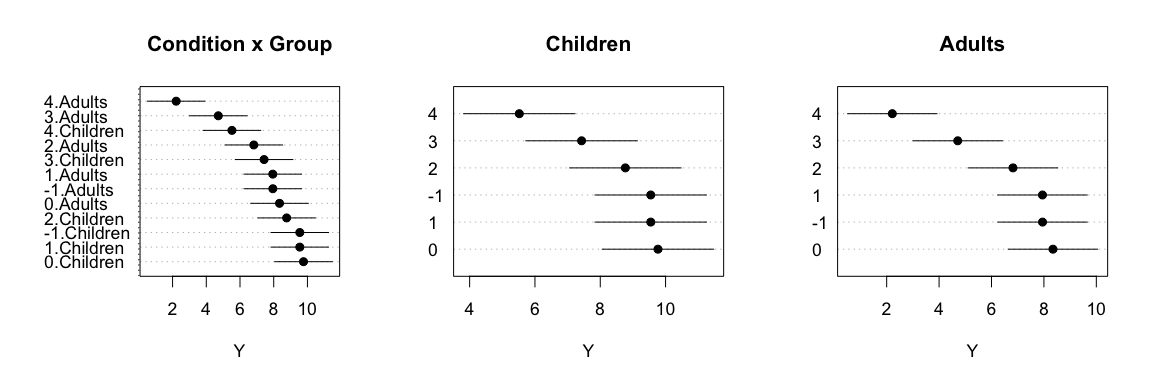## Inspection of partial effects

#### Function plot (mgcv)

par(mfrow=c(1,3), cex=1.1)
# first smooth of time:
abline(h=0)
# second smooth, of trial:
abline(h=0)
# third smooth, nonlienar interaction between Time and Trial:
plot(m1, select=3, scale=0, rug=FALSE)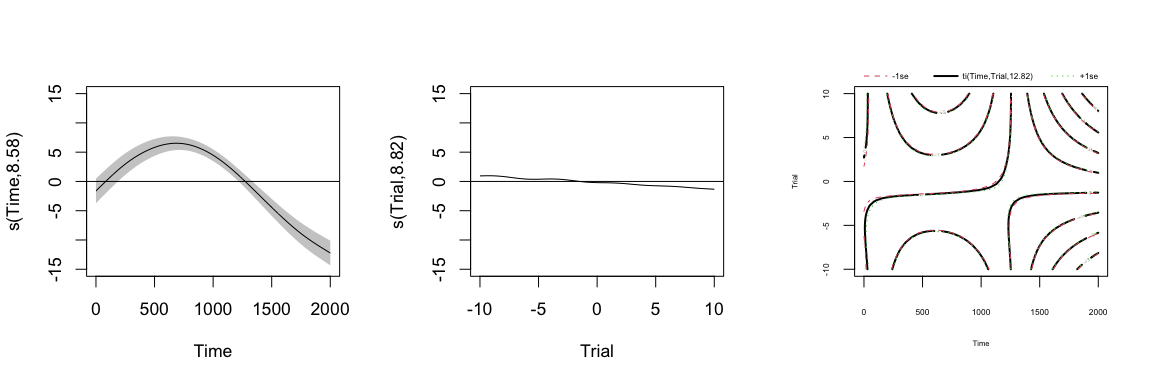#### Function pvisgam

The function pvisgam provides a more colorful version of the contour plot that visualizes the partial interaction between Time and Trial. The function can also plot two dimensional representations of higher order interactions.

Below a comparison:

par(mfrow=c(1,3), cex=1.1)
# Partial effect of nonlinear interaction between Time and Trial:
plot(m1, select=3, scale=0, rug=FALSE)
# Partial effect of nonlinear interaction between Time and Trial:
pvisgam(m1, select=3, view=c("Time", "Trial"), main="pvisgam", dec=1)
# Summed effect of nonlinear interaction between Time and Trial:
fvisgam(m1, view=c("Time", "Trial"), rm.ranef=TRUE, main="fvisgam", dec=1)
## Warning in gradientLegend(c(min.z, max.z), n.seg = 3, pos = 0.875, color =
## pal, : Increase right margin to fit labels or decrease the number of decimals,
## see help(gradientLegend).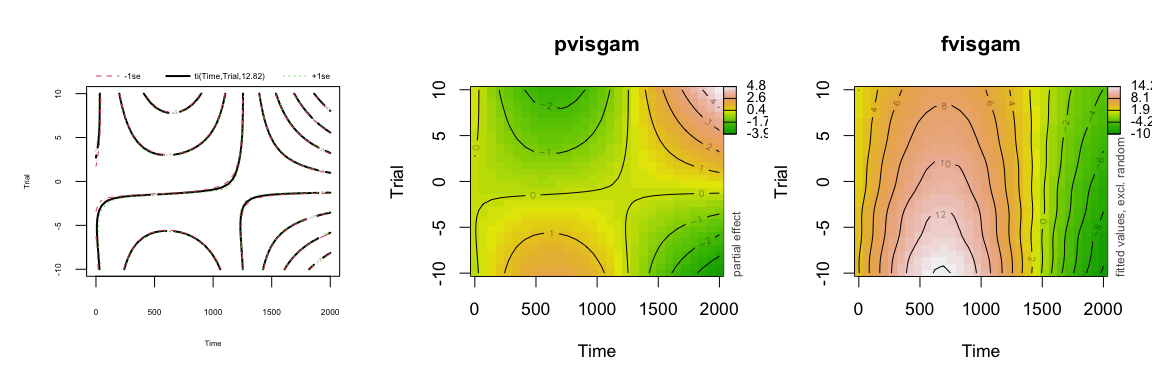#### Function inspect_random

The function plot plots also random smooths, just as the function inspect_random:

par(mfrow=c(1,2), cex=1.1)
plot(m1, select=4)
abline(h=0)
inspect_random(m1, select=4)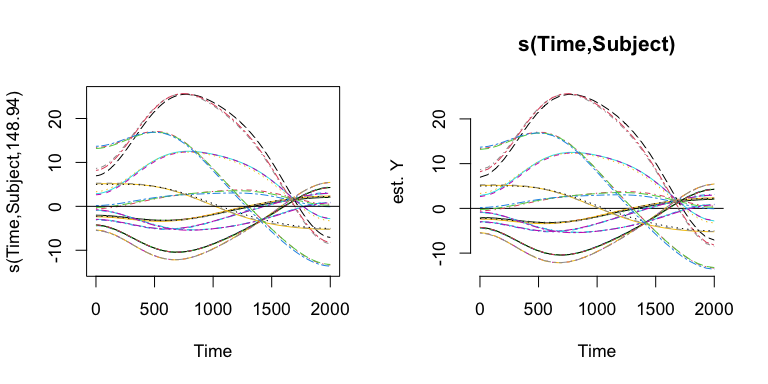The function inspect_random can also plot selective random effects, or apply a function over the random effects:

par(mfrow=c(1,2), cex=1.1)
# PLOT 1: selection
# ... the adults in black:
inspect_random(m1, select=4, cond=list(Subject=unique(simdat[simdat$Group=="Adults", "Subject"])), col=1, ylim=c(-15, 15), xpd=TRUE) # ... add children in red: inspect_random(m1, select=4, cond=list(Subject=unique(simdat[simdat$Group=="Children", "Subject"])), col=2, add=TRUE, xpd=TRUE)

# PLOT 2: averages
inspect_random(m1, select=4, fun=mean, ylim=c(-15,15), cond=list(Subject=unique(simdat[simdat$Group=="Adults", "Subject"])), lwd=2) # ... of the children: inspect_random(m1, select=4, fun=mean, cond=list(Subject=unique(simdat[simdat$Group=="Children", "Subject"])), lwd=2, col=2, add=TRUE)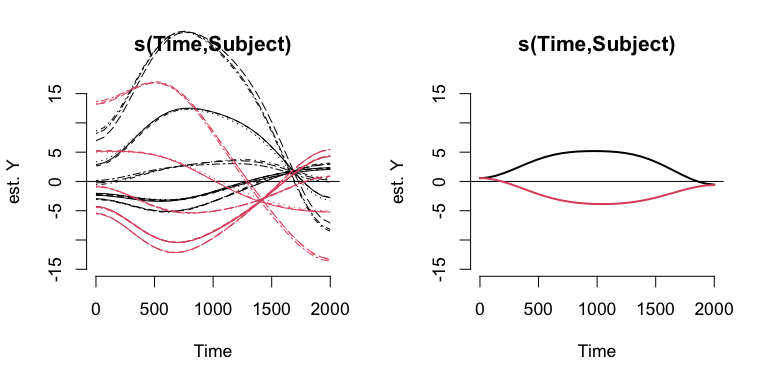Note that this last plot suggest that children and adults not oly differ overall, but also differ in their pattern over time. So it may be preferred to include the grouping predictor Group in the nonlinear ‘fixed’ (non-random) effects, to capture this difference in the fixed effects terms of the model.

## Other functions

#### Function plot_data

Inspect the data on which the model is based:

# All data, clustered by Group (very small dots):
plot_data(m1, view="Time", split_by="Group", cex=.5)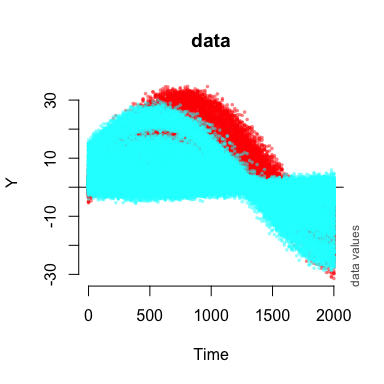#### Function plot_modelfit

Inspect the model fit with plot_modelfit:

simdat$Event <- interaction(simdat$Subject, simdat$Trial) plot_modelfit(m1, view="Time", event=simdat$Event, n=8)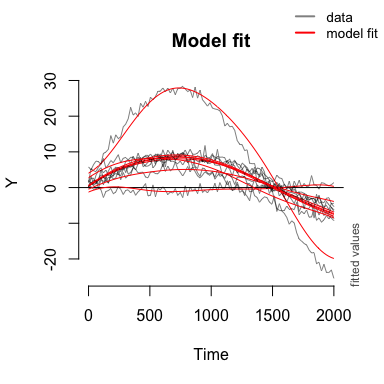#### Function plot_topo

The function plot_topo is a variant on the functions pvisgam and fvisgam for plotting EEG topographies. See the help file of plot_topo for more examples.

data(eeg)
# simple GAMM model:
m1 <- gam(Ampl ~ te(Time, X, Y, k=c(10,5,5),
d=c(1,2)), data=eeg)
# get electrode postions:
electrodes <- eeg[,c('X','Y','Electrode')]
electrodes <- as.list( electrodes[!duplicated(electrodes),] )
# topo plot, by default uses fvisgam
# and automatically selects a timestamp (270ms):
plot_topo(m1, view=c("X", "Y"), el.pos=electrodes, dec=2)